5.1.2. Neutrons in an External Field

For a neutron the Dirac Hamiltonian is somewhat simpler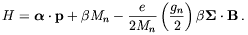(5.10)

with gn = -3.82. Again for pz = 0 the states of lowest energy, the ones we shall be interested in, have energies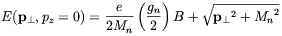(5.11)

Boosting to a finite pz we obtain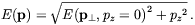(5.12)

The wave functions corresponding to this energy are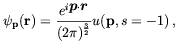(5.13)

where u(p, s = -1) is the standard spinor for a particle with momentum p, energy sqrt[p2 + Mn2] (not the energy of Eq. (5.12) and spin down.

In the non-relativistic limit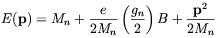(5.14)

and the wave functions are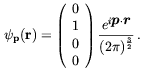(5.15)